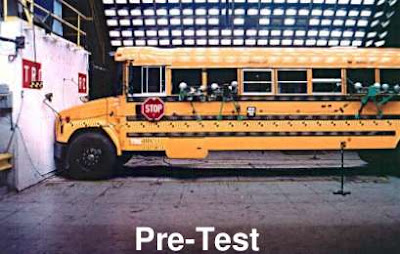## torsdag 10 september 2009

### Analytical vs Computational Mathematics, at KTH...Crash simulation of school mathematics.

In the Fall 2010 a new Bachelors Program in Simulation Technology will start at the Royal Institute of Technology KTH based on the Body&Soul Applied Mathematics Reform Program.

In the new B&S program standard analytical mathematics of calculus and linear algebra is combined with computational calculus and linear algebra into an integrated synthesis of analytical and computational mathematics, which opens entirely new possibilities in teaching and learning in science, technology, simulation, visualization and virtual reality.

This is because calculus and linear algebra boosted by computers gives a new very powerful tool allowing simulation of complex phenomena of real and imagined worlds unreachable by analytical mathematics.

The shift from standard analytical mathematics to computational mathematics has met strong opposition from the mathematics department at KTH unable or unwilling to reform standard analytical mathematics courses. The fact that KTH anyway has decided to start a program based on computational mathematics, given by people outside the mathematics department, opens to a similar reform in engineering education as a whole...

one can see the shift at KTH from analytical to computational mathematics as replacing standard analytical mathematics by IT, a shift which can propagate down through the whole school system....

The consequences of the KTH decision thus can be far-reaching, since KTH is a leading university and sets the agenda for school mathematics...in Sweden at least...